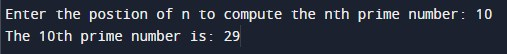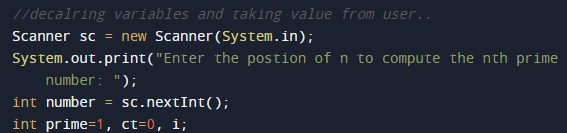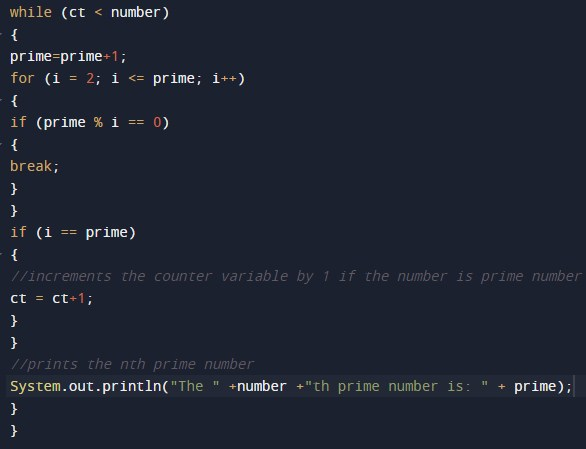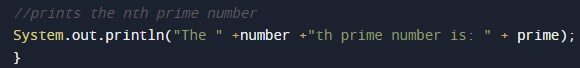# Program to check nth Prime Number In Java

In this tutorial you will learn about the Program to check nth Prime Number In Java and its application with practical example.

In this tutorial, we will learn to create a Java Program to check nth Prime Number In Java.

## Prerequisites

Before starting with this tutorial, we assume that you are best aware of the following Java programming concepts:

• Java Operators.
• Basic Input and Output function in Java.
• Class and Object in Java.
• Basic Java programming.
• If-else statements in Java.
• For loop in Java.

## Prime Number.

A number is called a prime if it is divisible by 1 or itself only.

Example:=>  2, 3, 5, 7, 11 and etc…

except  2 all are odd numbers.

## Program to check nth Prime Number In Java

In this program we would find the nth number position prime number.first of we would take a value from user and find the nth position prime number.let have a look at the code.

## OutputIn the above program, we have first declared and initialized a set variables required in the program.

• number = it will hold entered nth value number
• prime= it wil hold value of prime number
• i=  for iteration .
• ct= calculate the count value

After declaring variables we take a nth value of from user.and we will find the nth position prime number as shown in image below.## Approach to find nth Prime number.

we will used the same approach to find the prime number as w use to do always.

Steps for finding Prime nth Prime number.

• take an integer value (number) from the user.
• In loop,we execute the condition (ct<number). At Initially, set value “ct=0”.
• Increment the value “i” by 1.
• Check if the variable “i” is prime number or not.
• If true, increment the variable “ct” by “1”.
• follow this approach till nth number.
• and finally at last print the last prime number value.